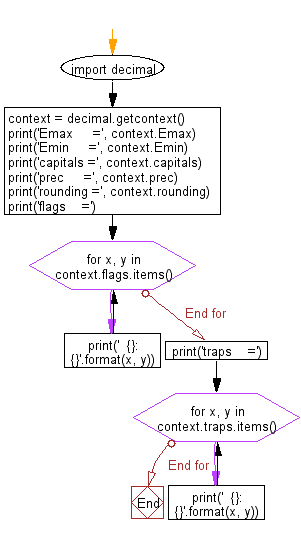﻿ Python Math: Retrieve the current global context for all decimal - w3resource# Python Math: Retrieve the current global context for all decimal

## Python Math: Exercise-39 with Solution

Write a Python program to retrieve the current global context (public properties) for all decimal.

Sample Solution:-

Python Code:

``````import decimal
context = decimal.getcontext()
print('Emax     =', context.Emax)
print('Emin     =', context.Emin)
print('capitals =', context.capitals)
print('prec     =', context.prec)
print('rounding =', context.rounding)
print('flags    =')
for x, y in context.flags.items():
print('  {}: {}'.format(x, y))
print('traps    =')
for x, y in context.traps.items():
print('  {}: {}'.format(x, y))
```
```

Sample Output:

```Emax     = 999999
Emin     = -999999
capitals = 1
prec     = 28
rounding = ROUND_HALF_EVEN
flags    =
<class 'decimal.InvalidOperation'>: False
<class 'decimal.FloatOperation'>: False
-----
<class 'decimal.Rounded'>: False
<class 'decimal.Clamped'>: False
```

Flowchart:Python Code Editor:

Have another way to solve this solution? Contribute your code (and comments) through Disqus.

What is the difficulty level of this exercise?

Test your Programming skills with w3resource's quiz.

﻿

```>>> students = [{'name': 'John', 'score': 98}, {'name': 'Mike', 'score': 94}, {'name': 'Jennifer', 'score': 99}]